Tamilnadu State Board New Syllabus Samacheer Kalvi 8th Maths Guide Pdf Chapter 2 Measurements Ex 2.3 Text Book Back Questions and Answers, Notes.

## Tamilnadu Samacheer Kalvi 8th Maths Solutions Chapter 2 Measurements Ex 2.3

Question 1.
Fill ini the blanks:

(i) The three dimensions of a cuboid are ______ , ______ and ______ .

(ii) The meeting point of more than two edges in a polyhedron is called as ______ .
Vertex(iii) A cube has _________ faces.
six

(iv) The cross section of a solid cylinder is ______ .
circle

(v) If a net of a 3-D shape has six plane squares, then it is called ______ .
cube

Question 2.
Match the following(i) – b
(ii) – a
(iii) – d
(iv) – cQuestion 3.
Which 3 – D shapes do the following nets represents? Draw them.
(i)The net represents cube, because it has 6 squares.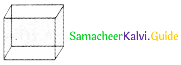(ii)The net represents cuboid(iii)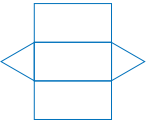The net represents Triangular prism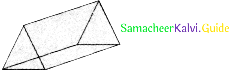(iv)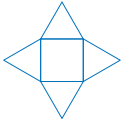The net represents square pyramid(v)Ine net represents cylinder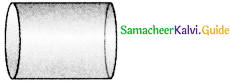Question 4.
For each solid, three views are given. Identify for each solid, the corresponding Top, Front and Side (T, F and S) views.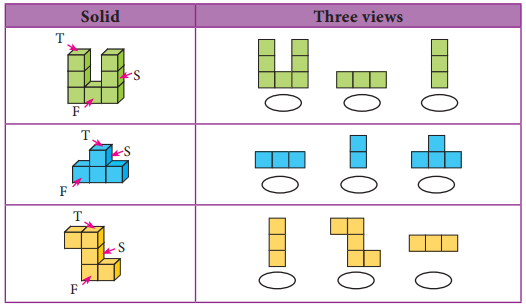Question 5.
Verify Euler’s formula for the table given below.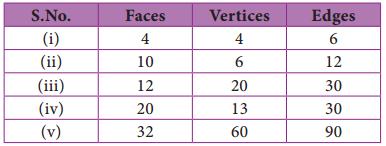Euler’s formula is given by F + V – E = 2
(i) F = 4; V = 4; E = 6
F + V – E = 4 + 4 – 6 = 8 – 6
F + V – E = 2
∴ Euler’s formula is satisfied.

(ii) F = 10; V = 6; E = 12
F + V – E = 10 + 6 – 12 = 16 – 12 = 4 ≠ 2
∴ Euler’s formula is not satisfied.

(iii) F = 12; V = 20; E = 30
F + V – E = 12 + 20 – 30 = 32 – 30 = 2
∴Euler’s formula is satisfied.

(iv) F = 20; V = 13; E = 30
F + V – E = 20 + 13 – 30 = 33 – 30 = 3 ≠ 2
∴Euler’s formula is not satisfied.

(v) F = 32; V = 60; E = 90
F + V – E = 32 + 60 – 90 = 92 – 90 = 2
∴ Euler’s formula is satisfied.